Question

1B. A baseball with a mass of LaTeX: 0.145 kg 0.145 k g is thrown to the left at a speed of LaTeX: 42.0 m/s 42.0 m / s. The ball is struck by a bat and leaves the bat with a speed of LaTeX: 49.0 m/s 49.0 m / s. If the time of the collision is LaTeX: 0.700 ms 0.700 m s, what is the average force of the bat on the ball? Neglect air resistance and assume the mass of the bat is so much larger than the ball that the bat does not rebound at all in the collision. Be careful with your signs. You must observe direction in this equation. What is the impulse of the bat on the ball? What is the momentum of the ball after it is hit by the bat?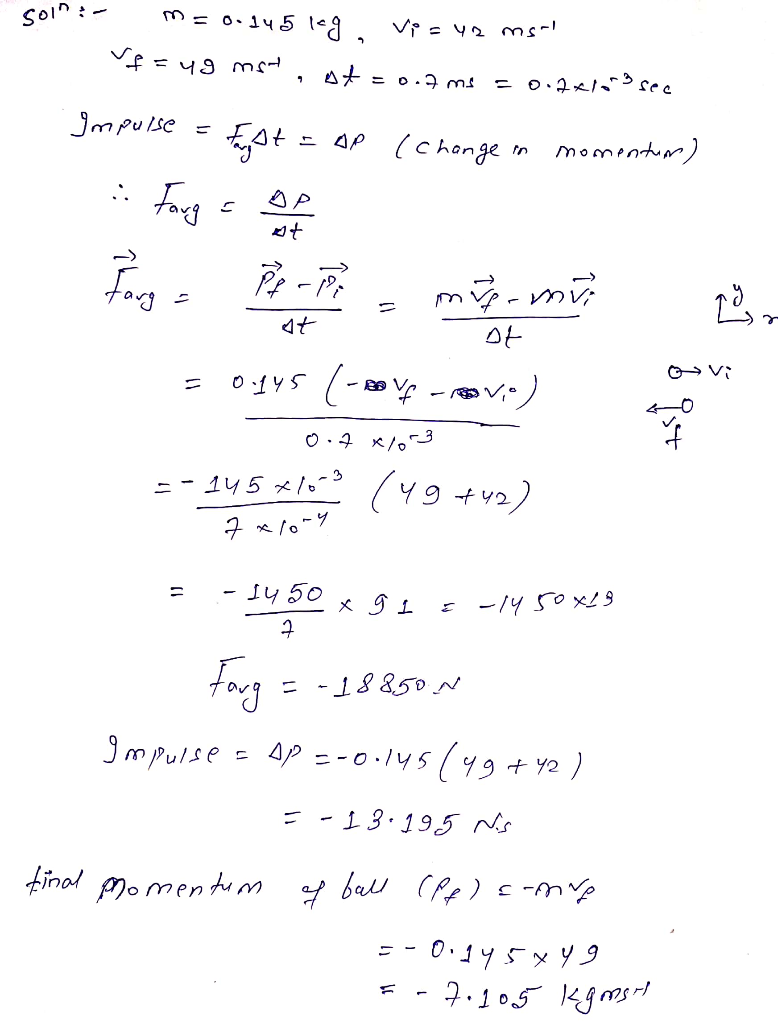#### Earn Coins

Coins can be redeemed for fabulous gifts.

Similar Homework Help Questions
• ### A baseball with a mass of 137 g is thrown horizontally with a speed of 40.4...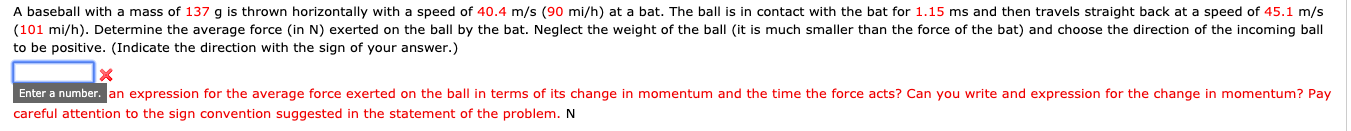A baseball with a mass of 137 g is thrown horizontally with a speed of 40.4 m/s (90 mi/h) at a bat. The ball is in contact with the bat for 1.15 ms and then travels straight back at a speed of 45.1 m/s (101 mi/h). Determine the average force in N) exerted on the ball by the bat. Neglect the weight of the ball (it is much smaller than the force of the bat) and choose the direction of...

• ### A baseball with a mass of 152 g is thrown horizontally with a speed of 40.7...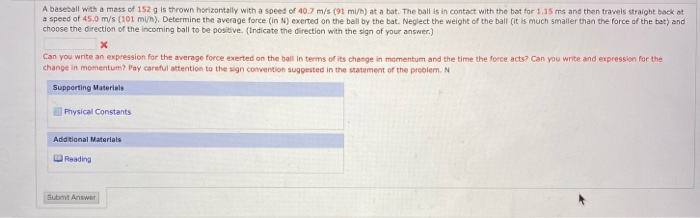A baseball with a mass of 152 g is thrown horizontally with a speed of 40.7 m/s (91 mi/h) at a bat. The ball is in contact with the bat for 1.15 ms and then travels straight back at a speed of 45.0 m/s (101 mi/h). Determine the average force (in N) exerted on the ball by the bat. Neglect the weight of the ball (it is much smaller than the force of the bat) and choose the direction of...

• ### A baseball with a mass of 141 g is thrown horizontally with a speed of 40.1...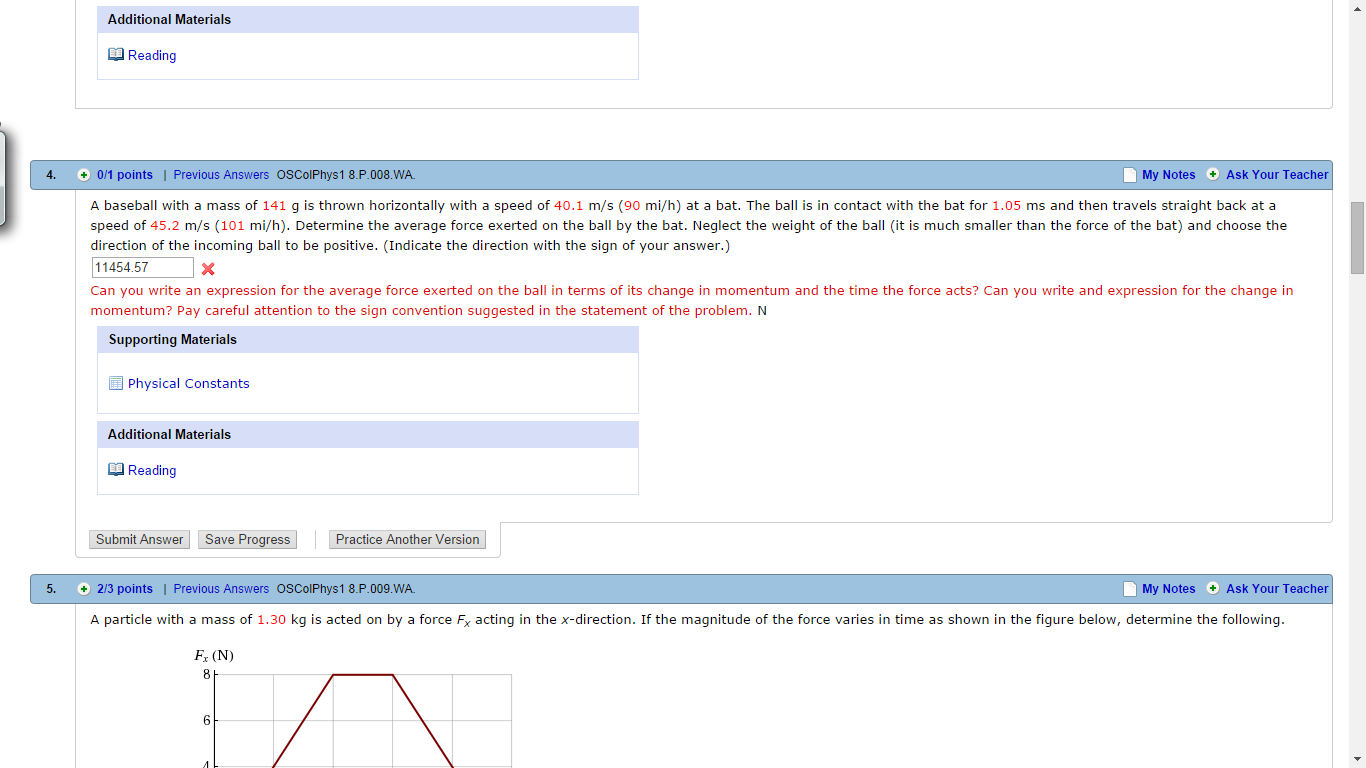A baseball with a mass of 141 g is thrown horizontally with a speed of 40.1 m/s (90 mi/h) at a bat. The ball is in contact with the bat for 1.05 ms and then travels straight back at a speed of 45.2 m/s (101 mi/h). Determine the average force exerted on the ball by the bat. Neglect the weight of the ball (it is much smaller than the force of the bat) and choose the direction of the incoming...

• ### A 0.145- kg baseball with an initial speed of 29 m/s rebounds with a speed of...

A 0.145- kg baseball with an initial speed of 29 m/s rebounds with a speed of 39 m/s after being struck with a bat. If the duration of contact between ball and bat was 1.6 ms, what was the magnitude of the average force between ball and bat?

• ### A 0.145- kg baseball with an initial speed of 25 m/s rebounds with a speed of...

A 0.145- kg baseball with an initial speed of 25 m/s rebounds with a speed of 35 m/s after being struck with a bat. If the duration of contact between ball and bat was 2.7 ms, what was the magnitude of the average force between ball and bat??

• ### (12) 12. A baseball with mase 145 12. A baseball with mass 145 g is thrown...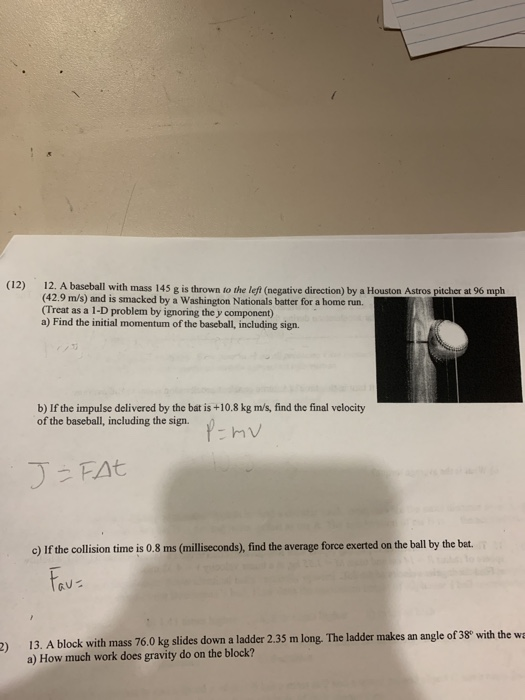(12) 12. A baseball with mase 145 12. A baseball with mass 145 g is thrown to the left (negative direction) by a Houston Astros pitcher at 96 mph (42.9 m/s) and is smacked by a Washington Nationals batter for a home run. (Treat as a 1-D problem by ignoring the y component) a) Find the initial momentum of the baseball, including sign. b) If the impulse delivered by the bat is +10.8 kg m/s, find the final velocity of...

• ### 8. A 0.145 kg baseball pitched at 35.0 m/s is hit on a horizontal line drive...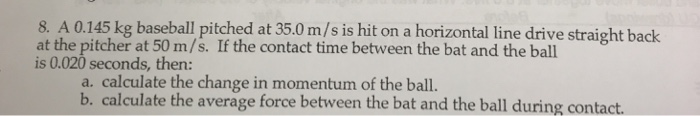8. A 0.145 kg baseball pitched at 35.0 m/s is hit on a horizontal line drive straight back at the pitcher at 50 m/s. If the contact time between the bat and the ball is 0.020 seconds, then: a. calculate the change in momentum of the ball. b. calculate the average force between the bat and the ball during contact.

• ### a .17 kg baseball is thrown w/ a initial speed of 38 m/s and it is hit straight back to the...

a .17 kg baseball is thrown w/ a initial speed of 38 m/s and it is hit straight back to the pitcher w/ a speed of 62 m/s. What is the magnitude of the IMPULSE exertedupon the ball by the bat?ans= 17 N*s

• ### A 156g baseball is thrown with a speed of 22 m/s. It is hit in a...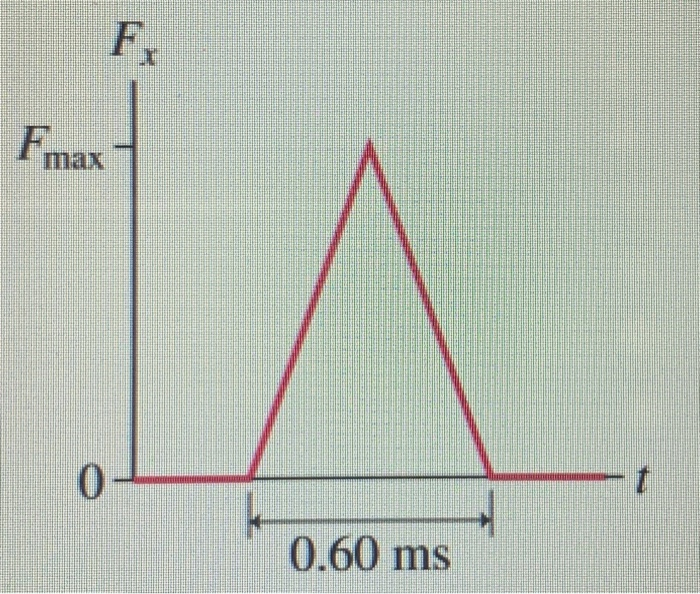A 156g baseball is thrown with a speed of 22 m/s. It is hit in a straight line back toward the pitcher at a speed of 37 m/s. The impulse force of the bat is shown. (a) What is the maximum force Fmax that the bat exerts on the ball? (b) What is the average force of the bat on the ball? Fx F max t 0 0.60 ms

• ### PRACTICE IT Use the worked example above to help you solve this problem. A golf ball...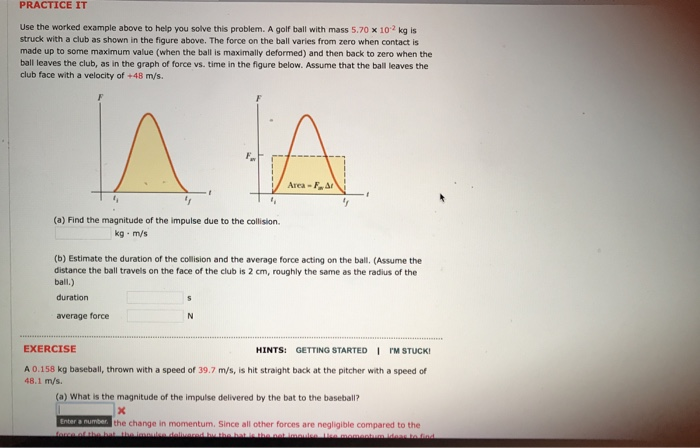PRACTICE IT Use the worked example above to help you solve this problem. A golf ball with mass 5.70 x 10 2 kg is struck with a club as shown in the figure above. The force on the ball varies from zero when contact is made up to some maximum value (when the ball is maximally deformed) and then back to zero when the ball leaves the club, as in the graph of force vs. time in the figure below....